PSA2RMA - Classwork---Geometry, M1, Lesson 7 (G.CO.9)
Part A)

Use the diagram below to determine x and y.andare straight lines.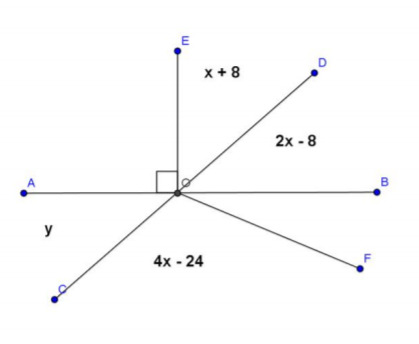x = ________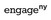Modified from EngageNY ©Great Minds Disclaimer

Type your answer below as a number (example: 5, 3.1, 4 1/2, or 3/2):
Part B)

y = ________

Type your answer below as a number (example: 5, 3.1, 4 1/2, or 3/2):
Part C)

Name a pair of vertical angles.

Part D)

Find the measure of ∠BOF. Do not include units in your answer.

Type your answer below as a number (example: 5, 3.1, 4 1/2, or 3/2):
Part E)

Part A)

Given lineand linein a plane, a third lineintersectsat a single point and intersectsat a single but different point. Lineand lineare parallel. Name the corresponding angles that are equal in measure.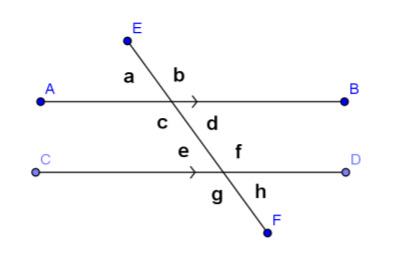Modified from EngageNY ©Great Minds Disclaimer

Part B)

Name the alternate interior angles that are equal in measure.

Part C)

Name the same-side interior angles that are supplementary.

Part A)

What is the measure of ∠a?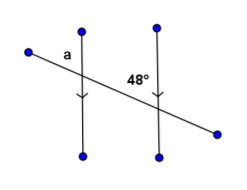Modified from EngageNY ©Great Minds Disclaimer

Type your answer below as a number (example: 5, 3.1, 4 1/2, or 3/2):
Part B)

What is the measure of ∠b?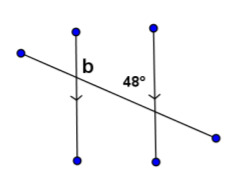Type your answer below as a number (example: 5, 3.1, 4 1/2, or 3/2):
Part C)

What is the measure of ∠c?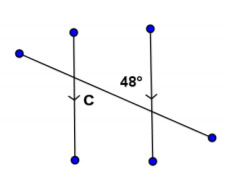Type your answer below as a number (example: 5, 3.1, 4 1/2, or 3/2):
Part D)

What is the measure of ∠d?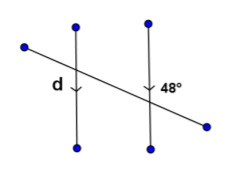Type your answer below as a number (example: 5, 3.1, 4 1/2, or 3/2):
Part A)

An ____________________ is sometimes useful when solving for unknown angles.Modified from EngageNY ©Great Minds Disclaimer

Part B)

In the figure below, we can use the auxiliary line to find the measures of ∠e and ∠f and then add the two measures together to find the measure of ∠W.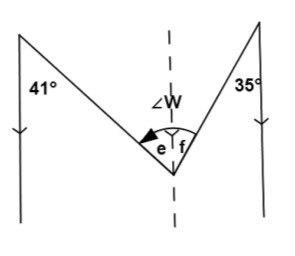= ________

Type your answer below as a number (example: 5, 3.1, 4 1/2, or 3/2):
Part C)= ________

Type your answer below as a number (example: 5, 3.1, 4 1/2, or 3/2):
Part D)= ________

Type your answer below as a number (example: 5, 3.1, 4 1/2, or 3/2):
Part A)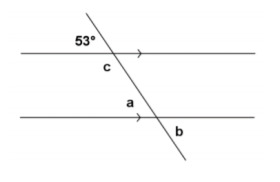= ________Modified from EngageNY ©Great Minds Disclaimer

Type your answer below as a number (example: 5, 3.1, 4 1/2, or 3/2):
Part B)

Give a reason for your solution.

Part C)= ________

Type your answer below as a number (example: 5, 3.1, 4 1/2, or 3/2):
Part D)

Give a reason for your solution.

Part E)= ________

Type your answer below as a number (example: 5, 3.1, 4 1/2, or 3/2):
Part F)

Give a reason for your solution.

Part A)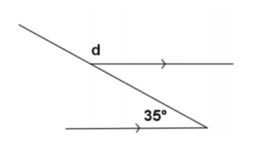= ________Modified from EngageNY ©Great Minds Disclaimer

Type your answer below as a number (example: 5, 3.1, 4 1/2, or 3/2):
Part B)

Give a reason for your solution.

Part A)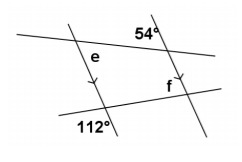= ________Modified from EngageNY ©Great Minds Disclaimer

Type your answer below as a number (example: 5, 3.1, 4 1/2, or 3/2):
Part B)

Give a reason for your solution.

Part C)= ________

Type your answer below as a number (example: 5, 3.1, 4 1/2, or 3/2):
Part D)

Give a reason for your solution.

Part A)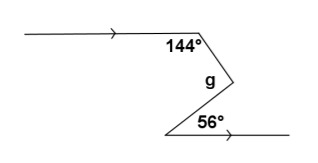= ________Modified from EngageNY ©Great Minds Disclaimer

Type your answer below as a number (example: 5, 3.1, 4 1/2, or 3/2):
Part B)

Give a reason for your solution.

Part A)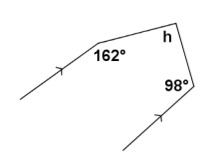= ________Modified from EngageNY ©Great Minds Disclaimer

Type your answer below as a number (example: 5, 3.1, 4 1/2, or 3/2):
Part B)

Give a reason for your solution.

Part A)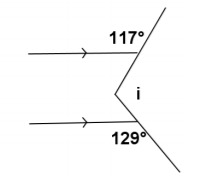= ________Modified from EngageNY ©Great Minds Disclaimer

Type your answer below as a number (example: 5, 3.1, 4 1/2, or 3/2):
Part B)

Give a reason for your solution.

Part A)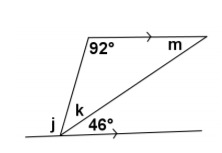= ________Modified from EngageNY ©Great Minds Disclaimer

Type your answer below as a number (example: 5, 3.1, 4 1/2, or 3/2):
Part B)

Give a reason for your solution.

Part C)= ________

Type your answer below as a number (example: 5, 3.1, 4 1/2, or 3/2):
Part D)

Give a reason for your solution.

Part E)= ________

Type your answer below as a number (example: 5, 3.1, 4 1/2, or 3/2):
Part F)

Give a reason for your solution.

Part A)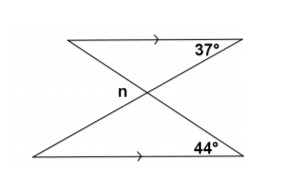= ________Modified from EngageNY ©Great Minds Disclaimer

Type your answer below as a number (example: 5, 3.1, 4 1/2, or 3/2):
Part B)

Give a reason for your solution.

Part A)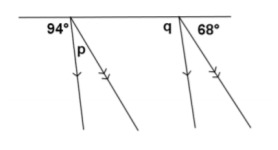= ________Modified from EngageNY ©Great Minds Disclaimer

Type your answer below as a number (example: 5, 3.1, 4 1/2, or 3/2):
Part B)

Give a reason for your solution.

Part C)= ________

Type your answer below as a number (example: 5, 3.1, 4 1/2, or 3/2):
Part D)

Give a reason for your solution.

Part A)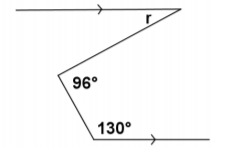= ________